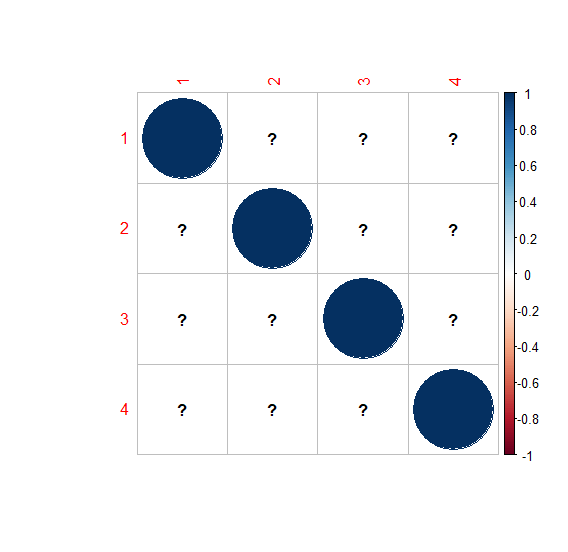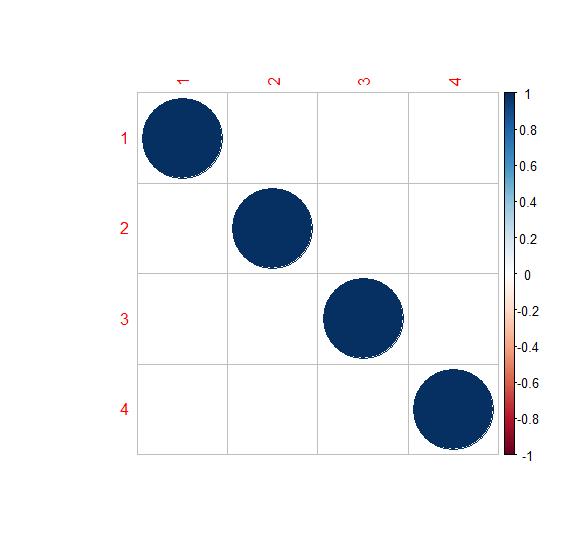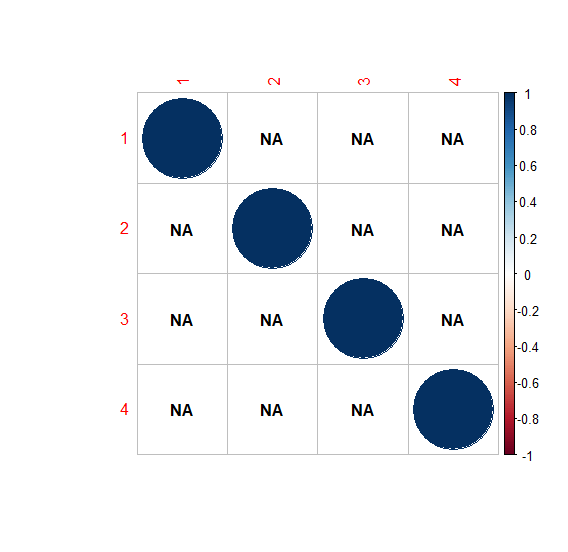# How to remove question mark from corrplot in R?

When we have NAs present in the data frame or matrix then correlation matrix contains NA values. Now if we create the correlation matrix plot using corrplot function the Output display question marks.

If we want to create the correlation matrix without question marks then we can use the na.label argument and set it to blank as shown in the below Example.

## Example

Following snippet creates a sample matrix −

M<-matrix(sample(c(NA,2,5,3),16,replace=TRUE),ncol=4)
M

The following matrix is created −

    [,1] [,2] [,3] [,4]
[1,] 3    2    5   NA
[2,] NA  NA    5    2
[3,] NA   2    5    2
[4,] 3    2   NA    2

To remove the question mark from the corrplot in R, on the above created data frame, add the following code to the above snippet −

M<-matrix(sample(c(NA,2,5,3),16,replace=TRUE),ncol=4)
M_corr<-cor(M)
M_corr

## Output

If you execute all the above given snippets as a single program, it generates the following Output −

    [,1] [,2]   [,3] [,4]
[1,]  1    NA    NA   NA
[2,] NA     1    NA   NA
[3,] NA    NA     1   NA
[4,] NA    NA    NA    1

To remove the question mark from the corrplot in R, on the above created data frame, add the following code to the above snippet −

M<-matrix(sample(c(NA,2,5,3),16,replace=TRUE),ncol=4)
M_corr<-cor(M)
corrplot(M_corr)

## Output

If you execute all the above given snippets as a single program, it generates the following Output −To remove the question mark from the corrplot in R, on the above created data frame, add the following code to the above snippet −

M<-matrix(sample(c(NA,2,5,3),16,replace=TRUE),ncol=4)
M_corr<-cor(M)
corrplot(M_corr,na.label=" ")

## Output

If you execute all the above given snippets as a single program, it generates the following Output −We can also replace question mark with NA as shown below −

M<-matrix(sample(c(NA,2,5,3),16,replace=TRUE),ncol=4)
M_corr<-cor(M)
corrplot(M_corr,na.label="NA")

## Output

If you execute all the above given snippets as a single program, it generates the following Output −